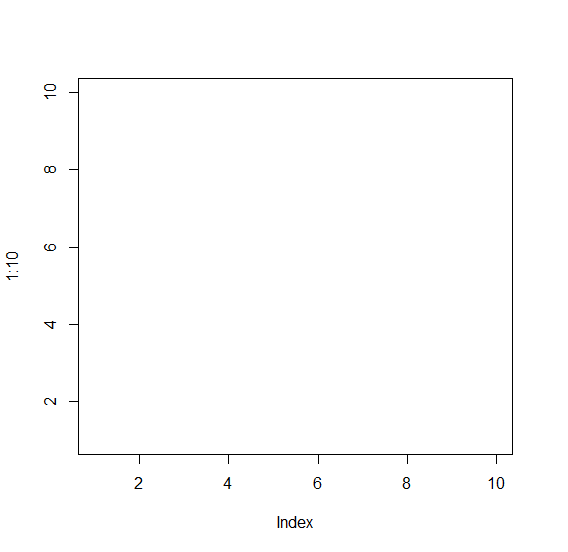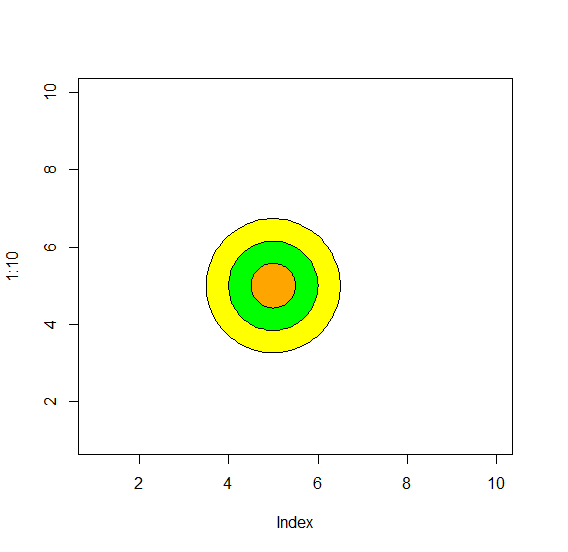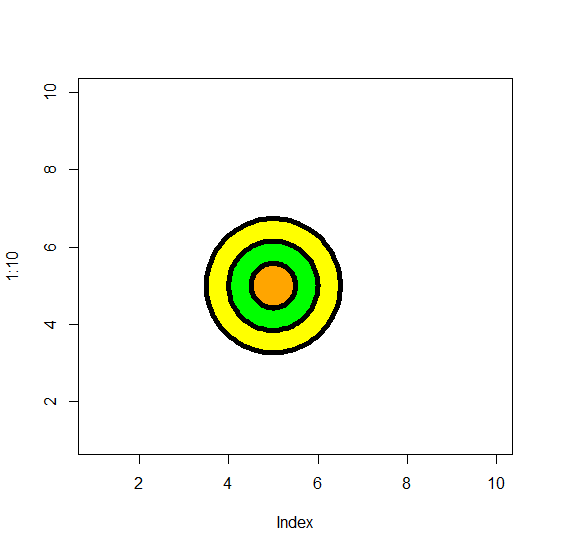How to draw concentric circles with dark borders in R?

R ProgrammingServer Side ProgrammingProgramming

To draw concentric circles, we can use draw.circle function of plotrix package where we can put lwd argument but firstly we would need to create a blank graph with plot function as shown below.

For Example, we can create three concentric circles at position X=5 and Y=5 having radius of 1, 2, and 3 by using the below command −

draw.circle(5,5,c(1.5,1,0.5),col=c("yellow","green","orange"),lwd=2)

Example

Consider the following snippet −

plot(1:10,type="n")

Output

If you execute the above given snippet, it generates the following Output −Add the following code to the above snippet −

plot(1:10,type="n")
library(plotrix)
draw.circle(5,5,c(1.5,1,0.5),col=c("yellow","green","orange"))

Output

If you execute all the above given snippets as a single program, it generates the following Output −Add the following code to the above snippet −

plot(1:10,type="n")
library(plotrix)
draw.circle(5,5,c(1.5,1,0.5),col=c("yellow","green","orange"))
draw.circle(5,5,c(1.5,1,0.5),col=c("yellow","green","orange"),lwd=2)

Output

If you execute all the above given snippets as a single program, it generates the following Output −Add the following code to the above snippet −

plot(1:10,type="n")
library(plotrix)
draw.circle(5,5,c(1.5,1,0.5),col=c("yellow","green","orange"))
draw.circle(5,5,c(1.5,1,0.5),col=c("yellow","green","orange"),lwd=2)
draw.circle(5,5,c(1.5,1,0.5),col=c("yellow","green","orange"),lwd=5)

Output

If you execute all the above given snippets as a single program, it generates the following Output −